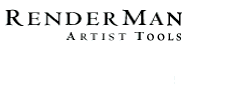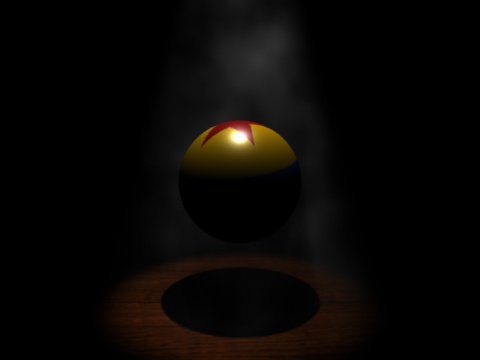PhotoRealistic RenderMan
Application Note #20

March, 1997

## Introduction

Starting with PhotoRealistic RenderMan 3.7, it is possible to sample lights from within a volume shader. This allows volume effects such as atmospheric haze, smoke, atmospheric shadows, etc. This Applications Note explains the general strategy for writing shaders to produce these effects, and lists a sample shader which implements a smoky appearance.

## General Strategy

The general idea behind the smoke effects is to ray march along the incident ray I, sampling illumination and accounting for atmospheric extinction. Typically, this is done with the following algorithm:

```	Choose an appropriate step size for marching along the ray.
total_len = length(I)
current_pos = P;
while total_len > 0 do:
sample the smoke density and light at current_pos
adjust Ci/Oi to add new light and extinguish due to smoke opacity.
current_pos += stepsize * normalize(-I);
total_len -= stepsize;
endwhile
```

Volume shaders of this type can be very expensive. The computational expense is proportional to the number of iterations of the while loop, which is determined by the step size and the length of I. Therefore, it is important to choose your stepsize carefully — too large a stepsize will result in banding and quantization artifacts, while too small a stepsize results in very long render times. You will probably need to carefully tune the stepsize on a scene-by-scene basis.

Also remember that atmosphere shaders bind to surfaces, just like surface or displacement shaders. So you must have an object in the background for the atmosphere shader to run. In other words, pixels with no geometry at all "behind" them will not run any atmosphere shaders.

Below is a shader which implements such a ray marching algorithm to simulate smoke. If the light sources in the scene cast shadows, you should be able to see the shadows in the smoke.

```/**************************************************************************
* smoke.sl
*
* Description:
*    This is a volume shader for smoke.  Trapezoidal integration is
*    used to integrate to find scattering and extinction.
*
* Parameters:
*   density - overall smoke density control
*   integstart, integend - bounds along the viewing ray direction of the
*          integration of atmospheric effects.
*   stepsize - step size for integration.  Note that the overall speed
*          of this shader is inversely proportional to the stepsize.
*   use_noise - makes the smoke noisy (nonuniform) when nonzero
*   freq, octaves, smokevary - control the fBm of the noisy smoke
*   lightscale - multiplier for light scattered toward viewer in volume
*   debug - if nonzero, copious output will be sent to stderr.
*
**************************************************************************/

#define snoise(p) (2*noise(p)-1)

volume
smoke (float density = 60;
float integstart = 0, integend = 1000;
float stepsize = 0.1;
float debug = 0;
float use_noise = 1;
color scatter = 1;   /* for sky, try (1, 2.25, 21) */
float octaves = 3, freq = 1, smokevary = 1;
float lightscale = 15;
)
{
point Worigin = P - I;
vector incident = vtransform ("shader", I);
point origin = transform ("shader", Worigin);
vector IN, WIN;
float tau;
color Cv = 0, Ov = 0;           /* net color & opacity of volume */
color dC, dO;                   /* differential color & opacity */
float ss, dtau, last_dtau;
color li, last_li, lighttau;
color scat;
point PP, PW, Psmoke;
float smoke, f, i;

float end = min (length (incident), integend) - 0.0001;

/* Integrate forwards from the start point */
float d = integstart + random()*stepsize;
if (d < end) {
IN = normalize (incident);
WIN = vtransform ("shader", "current", IN);
dtau = 0;
li = 0;
ss = min (stepsize, end-d);
d += ss;

while (d <= end) {
PP = origin + d*IN;
PW = Worigin + d*WIN;
last_dtau = dtau;
last_li = li;

li = 0;
illuminance (PW, vector(0,0,1), PI) { li += Cl; }
if (use_noise != 0) {
Psmoke = PP*freq;
smoke = snoise (Psmoke);
/* Optimize: one octave only if not lit */
if (comp(li,0)+comp(li,1)+comp(li,2) > 0.01) {
f = 1;
for (i=1;  i < octaves;  i+=1) {
f *= 0.5;  Psmoke *= 2;
smoke += f*snoise(Psmoke);
}
}
dtau = density * smoothstep(-1,1,smokevary*smoke);
} else dtau = density;

/* Our goal now is to find dC and dO, the color and opacity
* of the portion of the volume covered by this step.
*/
tau = .5 * ss * (dtau + last_dtau);
lighttau = .5 * ss * (li*dtau + last_li*last_dtau);

scat = -tau * scatter;
dO = 1 - color (exp(comp(scat,0)), exp(comp(scat,1)), exp(comp(scat,2)));
dC = lighttau * dO;

/* Now we adjust Cv/Ov to account for dC and dO */
Cv += (1-Ov)*dC;
Ov += (1-Ov)*dO;

ss = max (min (ss, end-d), 0.005);
d += ss;
}
}

Ci = lightscale*Cv + (1-Ov)*Ci;
Oi = Ov + (1-Ov)*Oi;
}
```

The image below was produced with this very shader: